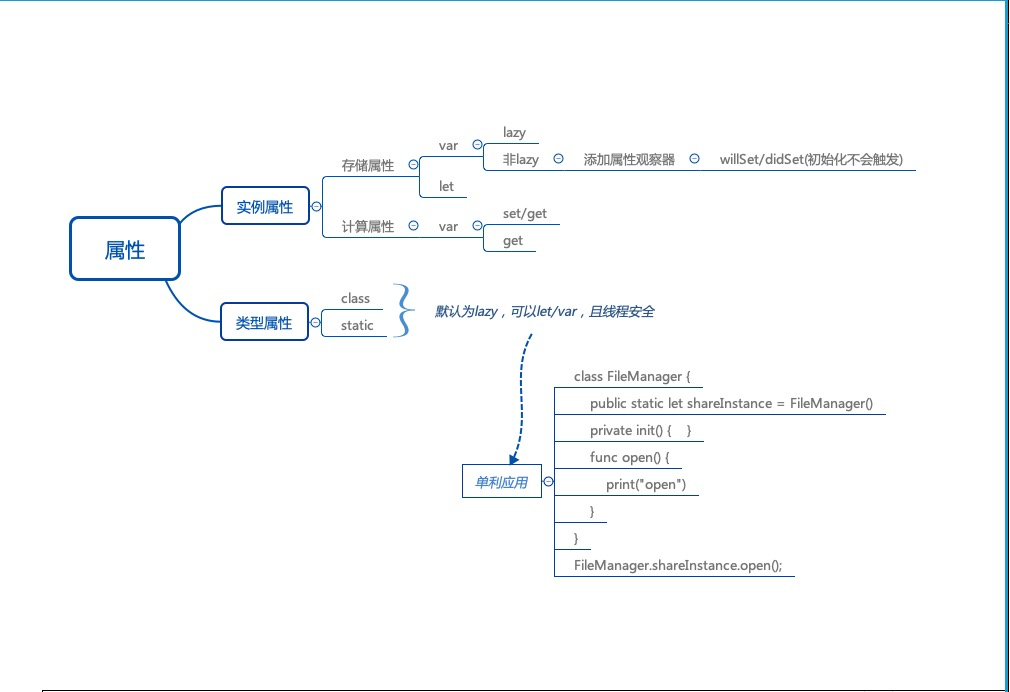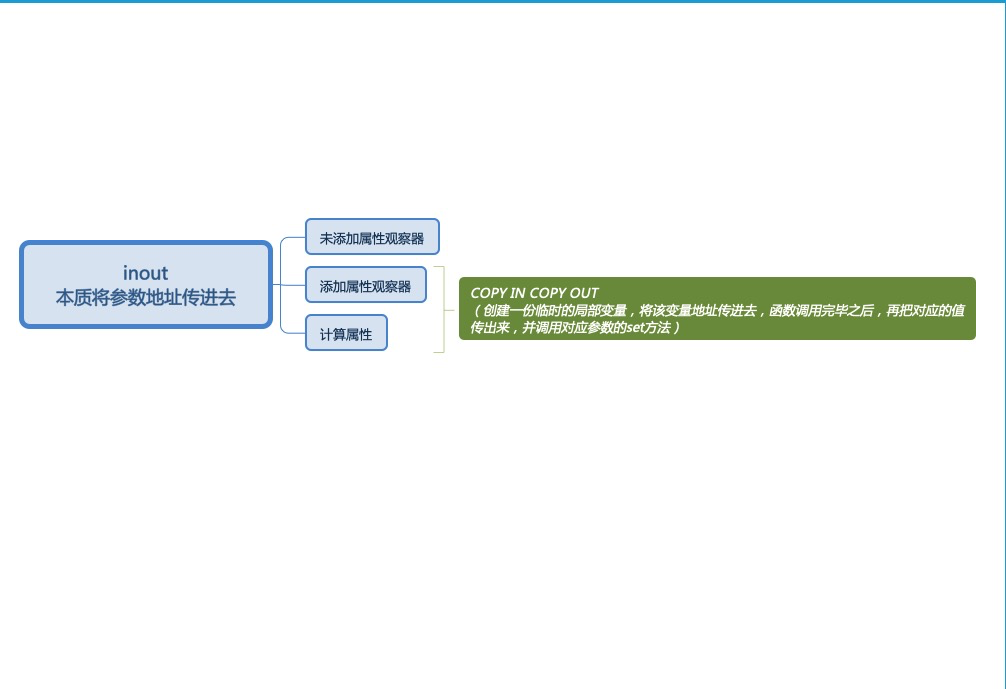2016-01-29 20:35:54 BaiHuaXiu123 阅读数 4116

1 double的格式化输出 例如保留两位小数
2 swift 的反射机制

`````` 1 double的格式化输出 例如保留两位小数
//Double的延展
extension Double {
func format(f: String) -> String {
return String(format: "%\(f)f", self)
}
}
let b:Double = 1.2568
let form = String(format: "%.2f",b)
let f = ".2f"
print("\(b.format(f))")
``````2 swift 的反射机制

``````接下来介绍一下swift的反射机制
Objective-C 中我们不太会经常提及到 “反射” 这样的词语，因为 Objective-C 的运行时比一般的反射还要灵活和强大。可能很多读者已经习以为常的像是通过字符串生成类或者 selector，并且进而生成对象或者调用方法等，其实都是反射的具体的表现。而在 Swift 中其实就算抛开 Objective-C 的运行时的部分，在纯 Swift 范畴内也存在有反射相关的一些内容，只不过相对来说功能要弱得多。

Swift 中所有的类型都实现了 _Reflectable，这是一个内部接口，我们可以通过 _reflect 来获取任意对象的一个镜像，这个镜像对象包含类型的基本信息，在 Swift 2.0 之前，这是对某个类型的对象进行探索的一种方法。在 Swift 2.0 中，这些方法已经从公开的标准库中移除了，取而代之，我们可以使用 Mirror 来
struct Person {
let name: String
let age: Int
}

let xiaoMing = Person(name: "XiaoMing", age: 16)
let r = Mirror(reflecting: xiaoMing) // r 是 MirrorType

print("xiaoMing 是 \(r.displayStyle!)")

print("属性个数:\(r.children.count)")
for i in r.children.startIndex..<r.children.endIndex {
print("属性名:\(r.children[i].0!)，值:\(r.children[i].1)")
}
``````

double输出 swift 相关内容

2019-09-12 12:49:41 songzhuo1991 阅读数 49
• Swift中跟实例相关的属性可分为两大类
• 存储属性：
1. 类似于成员变量这个概念
2. 存储在实力的内存中
3. 结构体、类可以定义存储属性
4. 枚举不可以定义存储属性
• 计算属性
1. 本质就是方法（函数）
2. 不占用实力的内存
3. 枚举、结构体、类都可以定义计算属性
``````struct Circel {
// 存储属性
// 计算属性
var diameter: Double {
set {
}
get {
}
}
}
print(circle.diameter) // 10.0
circle.diameter = 12
print(circle.diameter) // 12.0
print(MemoryLayout<Circel>.stride) // 8
``````

## 存储属性

• 关于存储属性，Swift有个明确的规定
• 在创建类 或 结构体的实例时，必须为所有的存储属性设置一个合适的初始值
1. 可以在初始化器里为存储属性设置一个初始值
2. 可以分配一个默认的属性值作为属性定义的一部分

## 计算属性

• set传入的新值默认叫做newValue，也可以自定义
``````struct Circle {
var diameter: Double {
set(newDiameter) {
}
get {
}
}
}
``````
• 只读计算属性：只有get，没有set
``````struct Cirecle {
var diameter: Double {
get {
}
}
}
``````
``````struct Cirecle {
var diameter: Double { radius * 2 }
}
``````
• 定义计算属性只能用var，不能有let
• let代表常量：值yichengbu的
• 计算属性的值是可能发生变化的（即使是只读计算属性）

## 枚举rawValue的原理

• 枚举原始值rawValue的本质是：只读计算属性
``````enum TestEnum: Int {
case test1 = 1, test2 = 2, test3 = 3
var rawValue: Int {
switch self {
case .test1:
return 10
case .test2:
return 11
case .test3:
return 12
}
}
}
print(TestEnum.test3.rawValue) // 12
``````

## 延迟存储属性（Lazy Stored Property）

• 使用lazy可以定义一个延迟存储属性，在第一次用到属性的时候才会进行初始化
``````class Car {
init() {
print("Car init")
}
func run() {
print("Car is runnig!")
}
}
class Person {
lazy var car = Car()
init() {
print("Person init!")
}
func goOut() {
car.run()
}
}
let p = Person()
print("------")
p.goOut()
// Person init!
// ------
// Car init!
// Car is running!
``````
``````class PhotoView {
lazy var image: Image = {
let url = "https://www.xxx.com/xx.png"
let data = Data(url: url)
return Image(data: data)
}()
}
``````
• lazy属性必须是var，不能是let
• let必须在实力的初始化方法完成之前就拥有值
• 如果多线程同时第一次访问lazy属性
• 无法保证属性只被初始化1次

## 延迟存储属性注意点

• 当结构体包含一个延迟存储属性时，只有var才能访问延迟存储属性
• 因为延迟属性初始化时需要改变结构体的内存
``````struct Point {
var x = 0
var y = 0
lazy var z = 0
}
let p = Point()
print(p.z)  // ·Cannot use mutating getter on immutable value:'p' is a 'let' constant
``````

## 属性观察器（Property Observer）

• 可以给非lazy的var存储属性设置属性观察器
``````struct Cirecle {
willSet {
print("willSet", newValue)
}
didSet {
print("didSet", oldValue, redius)
}
}
init() {
print("Cirecle init!")
}
}
// Cirecle init!
var circle = Circle()
// willSet 10.5
// didSet 1.0 10.5
// 10.5
``````
• willSet会传递新值，默认叫newValue
• didSet会传递旧值，默认叫oldValue
• 在初始化器中设置属性值不会触发willSet和didSet
• 在属性定义时设置初始化值也不会触发willSet和didSet

## 全局变量、局部变量

• 属性观察器、计算属性的功能，同样可以应用在全局变量、局部变量身上
``````var num: Int {
get {
return 10
}
set {
print("setNum", newValue)
}
}
num = 11 // setNum 11
print(num) // 10
``````
``````func test() {
var age = 10 {
willSet {
print("willSet", newValue)
}
didSet {
print("didSet", oldValue, age)
}
}
age = 11
// willSet 11
// didSet 10 11
}
``````

## 输入输出参数inout

``````struct Shape {
var width: Int
var side: Int {
willSet {
print("willSetSide", newValue)
}
didSet {
print("didSetSide", oldValue, side)
}
}
var girth: Int {
set {
width = newValue / side
print("setGirth", newValue)
}
get {
print("getGirth")
return width * side
}
}
func show() {
print("width=\(width), side=\(side), girth=\(girth)")
}
}
``````
``````func test(_ num: inout Int) {
num = 20
}
var s = Shape(width: 10, side: 4)
test(&s.width)
s.show()
print("------")
test(&s.side)
s.show()
print("------")
test(&s.girth)
s.show()
// get Girth
// width=20, side=4, girth=80
// ------
// willSetSide 20
// didSetSide 4 20
// getGirth
// width=20, side=20, girth=400
// ------
// getGirth
// setGirth 20
// getGirth
// width=1, side=20, girth=20
``````

## inout的本质总结

• 如果实参是有物理内存地址，且没有设置属性观察器

• 直接将实参的内存地址传入函数（实参进行引用传递）

• 如果实参是计算属性 或 设置了属性观察器

• 采用了Copy In Copy Out的做法

1. 调用函数时，先复制实参的值，产生副本【get】
2. 将副本的内存地址传入函数（副本进行引用传递），在函数内部可以修改副本的值
3. 函数返回后，再将副本的值覆盖实参的值【set】
• 总结：inout的本质就是引用传递（地址传递）

## 类型属性（Type Property）

• 严格来说，属性可以分为实例属性和类型属性

• 实例属性（Instance Property）：只能通过实例去访问

1. 存储实例属性（Stored Instance Property）：存储在实例的内存中，每个实例都有一份
2. 计算实例属性（Computed Instance Property）
• 类型属性（Type Property）：只能通过类型去访问
1. 存储类型属性（Stored Tyep Property）：整个程序运行过程中，就只有一份内存（类似于全局变量）
2. 计算类型属性（Computed Type Property）
• 可以通过static定义类型属性
• 如果是类，也可以用关键字class
``````struct Car {
static var count: Int = 0
init() {
Car.count = Self.count + 1
}
}
let c1 = Car()
let c2 = Car()
let c3 = Car()
print(Car.count) // 3
``````

## 类型属性细节

• 不同于存储实例属性，你必须给存储类型属性设定初始值

• 因为类型没有想实例那样的init初始化器来初始化存储属性

• 存储类型属性默认就是lazy，会在第一次使用的时候才初始化

• 就算被多个线程同时访问，保证只会初始化一次，因为调用了dispatch_once 函数，所以是线程安全的

• 存储类型属性可以是let

• 枚举类型可以定义类型属性（存储类型属性、计算类型属性）

## 单利模式

``````public class  FileManager {
public static let shared = FileManager()
private init() {}
}
``````
``````public class FileManager {
public static let shared = {
//...
//...
return FileManger()
}()
private init() {}
}
``````
• 图解：double输出 swift 相关内容

2019-02-10 17:26:30 pipitongkw1 阅读数 99

``````情况1：
double d=0.5;
printf(".0lf\n",d);

output:
1

double d=-0.5
printf("%.0lf\n",d);

output:
-1
``````

``````1.
1)函数原型：
double ceil( double arg );
float ceil( float arg );
2)函数作用：
返回正好大于等于arg的整数（实际上是"整数.000000")
3)example：
floor(3.5)=3;
floor(-3.2)=-4

2.
1)函数原型：
double ceil( double arg );
float ceil( float arg );
2)函数作用：
返回正好小于等于arg的整数（实际上是"整数.000000")
3)example:
ceil(3.5)=4;
ceil(-3.5)=-3;
``````

``````double m;
1)cout<<(int)(m+0.5);
2)printf("%.0lf",m);``````

double输出 swift 相关内容

2018-06-20 08:50:21 LeeCSDN77 阅读数 566
```override func viewDidLoad() {

//类型转换运算符: is, as
//可以用类型转换检查实例类型, 或者一个类型是否服从协议

class MediaItem {
var name: String
init(name: String) {
self.name = name
}
}

class Movie: MediaItem {
var director: String
init(name: String, director: String) {
self.director = director
super.init(name: name)
}
}

class Song: MediaItem {
var artist: String
init(name: String, artist: String) {
self.artist = artist
super.init(name: name)
}
}
//数组中有 Movie Class 类型,也有 Song Class 类型, 都继承自MediaItem, 编译器推断为 MediaItem类型
let library = [
Movie(name: "Casablanca", director: "Michael Curtiz"),
Song(name: "Blue Suede Shoes", artist: "Elvis Presley"),
Movie(name: "Citizen Kane", director: "Orson Welles"),
Song(name: "The One And Only", artist: "Chesney Hawkes"),
Song(name: "Never Gonna Give You Up", artist: "Rick Astley")
]
// the type of "library" is inferred to be [MediaItem]

//1. 检查类型
var movieCount = 0
var songCount = 0

for item in library {
if item is Movie {
movieCount += 1
} else if item is Song {
songCount += 1
}
}

print("Media library contains \(movieCount) movies and \(songCount) songs")
// Prints "Media library contains 2 movies and 3 songs"

//2. 向下类型转换(Downcasting)
//一个 Class 类型的常量或变量, 可能指向子类的实例. 这种情况下可以使用 as? 或 as! 进行向下类型转换
for item in library {
if let movie = item as? Movie {
print("Movie: \(movie.name), dir. \(movie.director)")
} else if let song = item as? Song {
print("Song: \(song.name), by \(song.artist)")
}
}

//3. Any 和 AnyObject 的类型转换
//Any 可以代表任何类型的实例, 包括函数类型
//AnyObject 可以代表任何 Class 类型的实例
//能确定类型的时候,要尽量写具体类型

var things = [Any]()

things.append(0)
things.append(0.0)
things.append(42)
things.append(3.14159)
things.append("hello")
things.append((3.0, 5.0))
things.append(Movie(name: "Ghostbusters", director: "Ivan Reitman"))
things.append({ (name: String) -> String in "Hello, \(name)" })

for thing in things {
switch thing {
case 0 as Int:
print("zero as an Int")
case 0 as Double:
print("zero as a Double")
case let someInt as Int:
print("an integer value of \(someInt)")
case let someDouble as Double where someDouble > 0:
print("a positive double value of \(someDouble)")
case is Double:
print("some other double value that I don't want to print")
case let someString as String:
print("a string value of \"\(someString)\"")
case let (x, y) as (Double, Double):
print("an (x, y) point at \(x), \(y)")
case let movie as Movie:
print("a movie called \(movie.name), dir. \(movie.director)")
case let stringConverter as (String) -> String:
print(stringConverter("Michael"))
default:
print("something else")
}

let optionalNumber: Int? = 3
things.append(optionalNumber)        // Warning
things.append(optionalNumber as Any) // No warning
}

// zero as an Int
// zero as a Double
// an integer value of 42
// a positive double value of 3.14159
// a string value of "hello"
// an (x, y) point at 3.0, 5.0
// a movie called Ghostbusters, dir. Ivan Reitman
// Hello, Michael
}```

double输出 swift 相关内容

2016-05-06 10:07:00 Shangjie2310 阅读数 2

NSLog(@"float:%.2f",a);

// 输出：

// double:1.23

let format = String(format:"%.2f",a)

print("double:\(format)")

// 输出：

// double:1.23

extension Double {

func format(f: String) -> String {

return String(format: "%\(f)f", self)

}

}

let f = ".2"

print("double:\(b.format(f))")

double输出 swift 相关内容